## Excel NA Function

This post will guide you how to use Excel NA function with syntax and examples in Microsoft excel.

### Description

The Excel NA function returns the #N/A error value. And the #N/A is the error value that means “no values is available”. And the #N/A error value will be produced when a formula is not able to find a valid value.

The NA function is a build-in function in Microsoft Excel and it is categorized as an Information Function.

The NA function is available in Excel 2016, Excel 2013, Excel 2010, Excel 2007, Excel 2003, Excel XP, Excel 2000, Excel 2011 for Mac.

### Syntax

The syntax of the NA function is as below:

`= NA ( )`

There are no parameters or arguments for the NA function.

Note:

• You must include the empty parentheses with the function name. Otherwise, Microsoft Excel will not recognize it as a function.
• You can also type the value #N/A directly into a cell. The NA function is provided for compatibility with other spreadsheet programs.

## Excel TYPE Function

This post will guide you how to use Excel TYPE function with syntax and examples in Microsoft excel.

### Description

The Excel TYPE function returns the type of a value. So you can use the TYPE function to return an integer that represents the value’s type in Excel. For example, if the value is a number, The TYPE function returns 1, and if the value is a text value, then the function will return 2.

The TYPE function is a build-in function in Microsoft Excel and it is categorized as an Information Function.

The TYPE function is available in Excel 2016, Excel 2013, Excel 2010, Excel 2007, Excel 2003, Excel XP, Excel 2000, Excel 2011 for Mac.

### Syntax

The syntax of the TYPE function is as below:

`= TYPE (value)`

Where the TYPE function argument is:

• Value -This is a required argument. The value that you want to get the type. it can be a number, text, logical value, etc.
 If value is TYPE returns Number 1 Text 2 Logical value 4 Error value 16 Array 64

### Excel TYPE Function Example

The below examples will show you how to use Excel TYPE Function to get the type of a value.

#1 to get the type of the value in cell B1, using the following formula:

=TYPE(B1)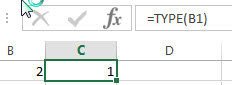#2 to get the type of the value “excelhow”, which is text, using the following formula:

= TYPE(“excelhow”)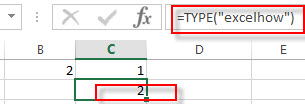3# to get the type of an array constant {2;3;5}, using the following formula:

= TYPE ({2;3;5})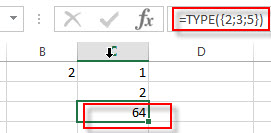## Excel N Function

This post will guide you how to use Excel N function with syntax and examples in Microsoft excel.

### Description

The Excel N function converts a value to a numeric value. And the N function can be used to convert the logical value TRUE as 1 and false value as 0. If you try to convert a text value, and the N function will return 0.

The N function is a build-in function in Microsoft Excel and it is categorized as an Information Function.

The N function is available in Excel 2016, Excel 2013, Excel 2010, Excel 2007, Excel 2003, Excel XP, Excel 2000, Excel 2011 for Mac.

### Syntax

The syntax of the N function is as below:

`=N(value)`

Where the N function argument is:

• Value – This is a required argument. The value that you want to convert.

N converts values listed in the following table.

 If value is or refers to N returns A number That number A date, in one of the built-in date formats available in Microsoft Excel The serial number of that date TRUE 1 FALSE 0 An error value, such as #DIV/0! The error value Anything else 0

### Excel N Function Example

The below examples will show you how to use Excel N Function to convert a value to a number.

#1 to convert the value in Cell B1 that contains a number, and the number will be returned, using the following formula:

`= N (B1)`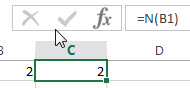#2 to convert the value in Cell B2 that contains text, and the zero will be returned, using the following formula:

`= N (B2)`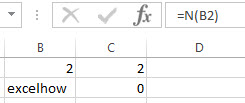#3 to convert the value in Cell B3 that contains a logical value TRUE, and 1 is returned, using the following formula:

`= N (B3)`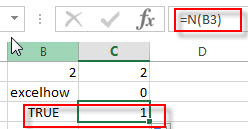### More Excel N Function Examples

• Count Number of Cells with String Length greater than X
You can create a new formula based on the SUMPRODUCT function, the N function and the LEN function to check the string length of each cell and also get the total number that the string length is greater than number 10.……

## Excel ISREF Function

This post will guide you how to use Excel ISREF function with syntax and examples in Microsoft excel.

### Description

The Excel ISREF function used to check if a value is a valid reference. If so, returns TRUE; if the value is not a reference, the function will return FALSE. So you can use the ISREF function to check for a value that is a reference in Excel.

The ISREF function is a build-in function in Microsoft Excel and it is categorized as an Information Function.

The ISREF function is available in Excel 2016, Excel 2013, Excel 2010, Excel 2007, Excel 2003, Excel XP, Excel 2000, Excel 2011 for Mac.

### Syntax

The syntax of the ISREF function is as below:

`=ISREF(value)`

Where the ISREF function argument is:

• Value -This is a required argument. The value that you want to test.

### Excel ISREF Function Example

The below examples will show you how to use Excel ISREF Function to check if a value is reference. And the ISREF function will return TRUE when the cell contains a valid reference.

#1 checks whether the value B1 is a valid reference, using the following formula:

`= ISREF (B1)`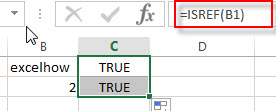### Related Functions

• Excel ISNUMBER Function
The Excel ISNUMBER function returns TRUE if the value in a cell is a numeric value, otherwise it will return FALSE.The syntax of the ISNUMBER function is as below:=ISNUMBER (value)
• Excel ISERR Function
The Excel ISERR function returns TRUE if the value is any error value except #N/A.The syntax of the ISERR function is as below:= ISERR (value)…
• Excel ISError Function
The Excel ISERROR function used to check for any error type that excel generates and it returns TRUE for any error type, such as: #N/A, #VALUE!,#REF!,#DIV0!, #NAME?, etc. The syntax of the ISERROR function is as below:= ISERROR (value)…
• Excel ISBlank Function
The Excel ISBLANK function returns TRUE if the value is blank or null.The syntax of the ISBLANK function is as below:=ISBLANK (value)…
• Excel ISNA Function
The Excel ISNA function used to check if a cell contains the #N/A error, if so, returns TRUE; otherwise, the ISNA function returns FALSE. The syntax of the ISNA function is as below:=ISNA(value)…
• Excel ISTEXT Function
The Excel ISTEXT function used to check if a value is text. If so, returns TRUE; if the text is not text, the function will return FALSE. The syntax of the ISTEXT function is as below:=ISTEXT(value)…
• Excel ISNONTEXT Function
The Excel ISNONTEXT function used to check if a value is text. If so, returns FALSE; if the text is not text, the function will return TRUE. The syntax of the ISNONTEXT function is as below:=ISNONTEXT (value)…

## Excel ISNONTEXT Function

This post will guide you how to use Excel ISNONTEXT function with syntax and examples in Microsoft excel.

### Description

The Excel ISNONTEXT function used to check if a value is text. If so, returns FALSE; if the text is not text, the function will return TRUE. So you can use the ISNONTEXT function to check for a value that is not text in Excel.

The ISNONTEXT function is a build-in function in Microsoft Excel and it is categorized as an Information Function.

The ISNONTEXT function is available in Excel 2016, Excel 2013, Excel 2010, Excel 2007, Excel 2003, Excel XP, Excel 2000, Excel 2011 for Mac.

### Syntax

The syntax of the ISNONTEXT function is as below:

`=ISNONTEXT (value)`

Where the ISNONTEXT function argument is:

• Value -This is a required argument. The value that you want to test.

### Excel ISNONTEXT Function Example

The below examples will show you how to use Excel ISNONTEXT Function to check if a value is not text. And the ISNONTEXT function will return TRUE when value is not a text string.

#1 checks whether the value in Cell B1 is not a text string, using the following formula:

= ISNONTEXT (B1)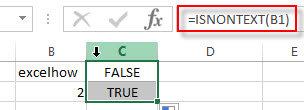### Related Functions

• Excel ISNUMBER Function
The Excel ISNUMBER function returns TRUE if the value in a cell is a numeric value, otherwise it will return FALSE.The syntax of the ISNUMBER function is as below:=ISNUMBER (value)
• Excel ISERR Function
The Excel ISERR function returns TRUE if the value is any error value except #N/A.The syntax of the ISERR function is as below:= ISERR (value)…
• Excel ISError Function
The Excel ISERROR function used to check for any error type that excel generates and it returns TRUE for any error type, such as: #N/A, #VALUE!,#REF!,#DIV0!, #NAME?, etc. The syntax of the ISERROR function is as below:= ISERROR (value)…
• Excel ISBlank Function
The Excel ISBLANK function returns TRUE if the value is blank or null.The syntax of the ISBLANK function is as below:=ISBLANK (value)…
• Excel ISNA Function
The Excel ISNA function used to check if a cell contains the #N/A error, if so, returns TRUE; otherwise, the ISNA function returns FALSE. The syntax of the ISNA function is as below:=ISNA(value)…
• Excel ISTEXT Function
The Excel ISTEXT function used to check if a value is text. If so, returns TRUE; if the text is not text, the function will return FALSE. The syntax of the ISTEXT function is as below:=ISTEXT(value)…
• Excel ISREF Function
The Excel ISREF function used to check if a value is a valid reference. If so, returns TRUE; if the value is not a reference, the function will return FALSE. The syntax of the ISREF function is as below:=ISREF(value)…

## Excel ISTEXT Function

This post will guide you how to use Excel ISTEXT function with syntax and examples in Microsoft excel.

### Description

The Excel ISTEXT function used to check if a value is text. If so, returns TRUE; if the text is not text, the function will return FALSE. So you can use the ISTEXT function to check for a value that is text in Excel.

The ISTEXT function is a build-in function in Microsoft Excel and it is categorized as an Information Function.

The ISTEXT function is available in Excel 2016, Excel 2013, Excel 2010, Excel 2007, Excel 2003, Excel XP, Excel 2000, Excel 2011 for Mac.

### Syntax

The syntax of the ISTEXT function is as below:

`=ISTEXT(value)`

Where the ISTEXT function argument is:

• Value -This is a required argument. The value that you want to test.

### Excel ISTEXT Function Example

The below examples will show you how to use Excel ISTEXT Function to check if a value is text. And the ISTEXT function will return TRUE when value is a text string.

#1 checks whether the value in Cell B1 is a text string, using the following formula:

`= ISTEXT (B1)`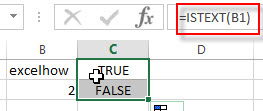### Related Functions

• Excel ISNUMBER Function
The Excel ISNUMBER function returns TRUE if the value in a cell is a numeric value, otherwise it will return FALSE.The syntax of the ISNUMBER function is as below:=ISNUMBER (value)
• Excel ISERR Function
The Excel ISERR function returns TRUE if the value is any error value except #N/A.The syntax of the ISERR function is as below:= ISERR (value)…
• Excel ISError Function
The Excel ISERROR function used to check for any error type that excel generates and it returns TRUE for any error type, such as: #N/A, #VALUE!,#REF!,#DIV0!, #NAME?, etc. The syntax of the ISERROR function is as below:= ISERROR (value)…
• Excel ISBlank Function
The Excel ISBLANK function returns TRUE if the value is blank or null.The syntax of the ISBLANK function is as below:=ISBLANK (value)…
• Excel ISNA Function
The Excel ISNA function used to check if a cell contains the #N/A error, if so, returns TRUE; otherwise, the ISNA function returns FALSE. The syntax of the ISNA function is as below:=ISNA(value)…
• Excel ISNONTEXT Function
The Excel ISNONTEXT function used to check if a value is text. If so, returns FALSE; if the text is not text, the function will return TRUE. The syntax of the ISNONTEXT function is as below:=ISNONTEXT (value)…
• Excel ISREF Function
The Excel ISREF function used to check if a value is a valid reference. If so, returns TRUE; if the value is not a reference, the function will return FALSE. The syntax of the ISREF function is as below:=ISREF(value)…

## Excel ISNA Function

This post will guide you how to use Excel ISNA function with syntax and examples in Microsoft excel.

### Description

The Excel ISNA function used to check if a cell contains the #N/A error, if so, returns TRUE; otherwise, the ISNA function returns FALSE. The #N/A error will be returned when a value is not available to your Excel formula.

The ISNA function is a build-in function in Microsoft Excel and it is categorized as an Information Function.

The ISNA function is available in Excel 2016, Excel 2013, Excel 2010, Excel 2007, Excel 2003, Excel XP, Excel 2000, Excel 2011 for Mac.

### Syntax

The syntax of the ISNA function is as below:

`=ISNA(value)`

Where the ISNA function argument is:

• Value – This is a required argument. The value that you want to test.

### Excel ISNA Function Example

The below examples will show you how to use Excel ISNA Function to text if a cell contain the #N/A error. For examples, if the Cell B1 contains #N/A error, the following ISNA formula will return TRUE.

`= ISNA(B1)`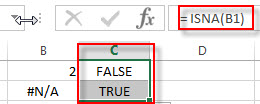### Related Functions

• Excel ISNUMBER Function
The Excel ISNUMBER function returns TRUE if the value in a cell is a numeric value, otherwise it will return FALSE.The syntax of the ISNUMBER function is as below:=ISNUMBER (value)
• Excel ISERR Function
The Excel ISERR function returns TRUE if the value is any error value except #N/A.The syntax of the ISERR function is as below:= ISERR (value)…
• Excel ISError Function
The Excel ISERROR function used to check for any error type that excel generates and it returns TRUE for any error type, such as: #N/A, #VALUE!,#REF!,#DIV0!, #NAME?, etc. The syntax of the ISERROR function is as below:= ISERROR (value)…
• Excel ISBlank Function
The Excel ISBLANK function returns TRUE if the value is blank or null.The syntax of the ISBLANK function is as below:=ISBLANK (value)…
• Excel ISTEXT Function
The Excel ISTEXT function used to check if a value is text. If so, returns TRUE; if the text is not text, the function will return FALSE. The syntax of the ISTEXT function is as below:=ISTEXT(value)…
• Excel ISNONTEXT Function
The Excel ISNONTEXT function used to check if a value is text. If so, returns FALSE; if the text is not text, the function will return TRUE. The syntax of the ISNONTEXT function is as below:=ISNONTEXT (value)…
• Excel ISREF Function
The Excel ISREF function used to check if a value is a valid reference. If so, returns TRUE; if the value is not a reference, the function will return FALSE. The syntax of the ISREF function is as below:=ISREF(value)…

### More Excel ISNA Function Examples

• Excel Vlookup Return True or False
you can use the VLOOKUP function to look for a value in a column in a table and then returns TRUE from a given column in that table if it finds something. If it doesn’t, it returns FALSE …

## Excel ISERR Function

This post will guide you how to use Excel ISERR function with syntax and examples in Microsoft excel.

### Description

The Excel ISERR function returns TRUE if the value is any error value except #N/A.

The ISERR function is a build-in function in Microsoft Excel and it is categorized as an Information Function.

The ISERR function is available in Excel 2016, Excel 2013, Excel 2010, Excel 2007, Excel 2003, Excel XP, Excel 2000, Excel 2011 for Mac.

### Syntax

The syntax of the ISERR function is as below:

= ISERR (value)

Where the ISERR function argument is:

• Value -This is a required argument. The value that you want to test.

### Example

The below examples will show you how to use Excel ISERR Function to test if the value is any of error values(except #N/A), if so, return TRUE.

#1 = ISERR (B1)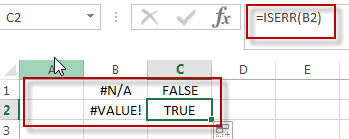### Related Functions

• Excel ISNUMBER Function
The Excel ISNUMBER function returns TRUE if the value in a cell is a numeric value, otherwise it will return FALSE.The syntax of the ISNUMBER function is as below:=ISNUMBER (value)
• Excel ISError Function
The Excel ISERROR function used to check for any error type that excel generates and it returns TRUE for any error type, such as: #N/A, #VALUE!,#REF!,#DIV0!, #NAME?, etc. The syntax of the ISERROR function is as below:= ISERROR (value)…
• Excel ISBlank Function
The Excel ISBLANK function returns TRUE if the value is blank or null.The syntax of the ISBLANK function is as below:=ISBLANK (value)…
• Excel ISNA Function
The Excel ISNA function used to check if a cell contains the #N/A error, if so, returns TRUE; otherwise, the ISNA function returns FALSE. The syntax of the ISNA function is as below:=ISNA(value)…
• Excel ISTEXT Function
The Excel ISTEXT function used to check if a value is text. If so, returns TRUE; if the text is not text, the function will return FALSE. The syntax of the ISTEXT function is as below:=ISTEXT(value)…
• Excel ISNONTEXT Function
The Excel ISNONTEXT function used to check if a value is text. If so, returns FALSE; if the text is not text, the function will return TRUE. The syntax of the ISNONTEXT function is as below:=ISNONTEXT (value)…
• Excel ISREF Function
The Excel ISREF function used to check if a value is a valid reference. If so, returns TRUE; if the value is not a reference, the function will return FALSE. The syntax of the ISREF function is as below:=ISREF(value)…

## ISNUMBER Function in Excel

This post will guide you how to use ISNUMBER function with syntax and examples in Microsoft excel.

## Description

The Excel ISNUMBER function returns TRUE if the value in a cell is a numeric value, otherwise it will return FALSE.

so you can use ISNUMBER function to check if a cell contains a numeric value or check the result of another function is a number in excel formula.

The ISNUMBER function is a build-in function in Microsoft Excel and it is categorized as an Information Function.

The ISNUMBER function is available in Excel 2016, Excel 2013, Excel 2010, Excel 2007, Excel 2003, Excel XP, Excel 2000,  Excel 2011 for Mac.

## Syntax

The syntax of the ISNUMBER function is as below:

`=ISNUMBER (value)`

Where the ISNUMBER function argument is:
Value -This is a required argument. The value that you want to test.

## ISNUMBER Function Examples

The below examples will show you how to use Excel ISNUMBER Function to test if a cell contains a numeric value, if so, return TRUE.

Example 1: check the value in cell B1 if it is a numeric value.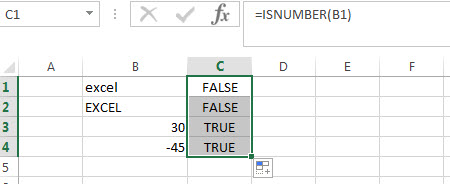#1 = ISNUMBER (B1)

It will return FALSE.

#2 =ISNUMBER (B3)

It will return TRUE.

### Excel ISNUMBER Function Examples

• Count Cells That Contain Specific Text
This post will discuss that how to count the number of cells that contain specific text or certain text in a specified cells of range in Excel. How to get the total number of cells that contain certain text.……
• Sort Cells by Specific word or words
how to sort cells or text values in a column based on a specific word even if the word is in the text string in the cell. How do I sort cells in a column by the number of a specific word or words in excel……

### Related Functions

• Excel ISERR Function
The Excel ISERR function returns TRUE if the value is any error value except #N/A.The syntax of the ISERR function is as below:= ISERR (value)…
• Excel ISError Function
The Excel ISERROR function used to check for any error type that excel generates and it returns TRUE for any error type, such as: #N/A, #VALUE!,#REF!,#DIV0!, #NAME?, etc. The syntax of the ISERROR function is as below:= ISERROR (value)…
• Excel ISBlank Function
The Excel ISBLANK function returns TRUE if the value is blank or null.The syntax of the ISBLANK function is as below:=ISBLANK (value)…
• Excel ISNA Function
The Excel ISNA function used to check if a cell contains the #N/A error, if so, returns TRUE; otherwise, the ISNA function returns FALSE. The syntax of the ISNA function is as below:=ISNA(value)…
• Excel ISTEXT Function
The Excel ISTEXT function used to check if a value is text. If so, returns TRUE; if the text is not text, the function will return FALSE. The syntax of the ISTEXT function is as below:=ISTEXT(value)…
• Excel ISNONTEXT Function
The Excel ISNONTEXT function used to check if a value is text. If so, returns FALSE; if the text is not text, the function will return TRUE. The syntax of the ISNONTEXT function is as below:=ISNONTEXT (value)…
• Excel ISREF Function
The Excel ISREF function used to check if a value is a valid reference. If so, returns TRUE; if the value is not a reference, the function will return FALSE. The syntax of the ISREF function is as below:=ISREF(value)…

## Excel ISError Function

This post will guide you how to use Excel ISERROR function with syntax and examples in Microsoft excel.

## Description

The Excel ISERROR function used to check for any error type that excel generates and it returns TRUE for any error type, such as: #N/A, #VALUE!,#REF!,#DIV0!, #NAME?, etc. and the ISERR function also can be checked for error values except #N/A error, it returns TRUE while the error is #N/A.

The ISERROR function is a build-in function in Microsoft Excel and it is categorized as an Information Function.

The ISERROR function is available in Excel 2016, Excel 2013, Excel 2010, Excel 2007, Excel 2003, Excel XP, Excel 2000, Excel 2011 for Mac.

## Syntax

The syntax of the ISERROR function is as below:

`= ISERROR (value)`

Where the ISERROR function argument is:

• Value -This is a required argument. The value that you want to test.

Example

The below examples will show you how to use Excel ISERROR Function to test if the value is any of error values. If so, return TRUE.

#1 using the following formula to check #N/A error.

`= ISERROR (B1)`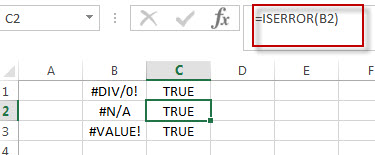#2 using the ISERROR function to check Cell B1 contains the value “excel”, enter the below formula in Cell C1 formula box:

`=ISERROR(B1)`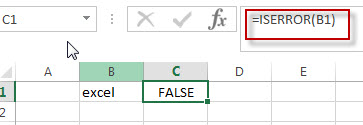As it is not an error type, so returns FALSE.

### Related Functions

• Excel ISNUMBER Function
The Excel ISNUMBER function returns TRUE if the value in a cell is a numeric value, otherwise it will return FALSE.The syntax of the ISNUMBER function is as below:=ISNUMBER (value)
• Excel ISERR Function
The Excel ISERR function returns TRUE if the value is any error value except #N/A.The syntax of the ISERR function is as below:= ISERR (value)…
• Excel ISBlank Function
The Excel ISBLANK function returns TRUE if the value is blank or null.The syntax of the ISBLANK function is as below:=ISBLANK (value)…
• Excel ISNA Function
The Excel ISNA function used to check if a cell contains the #N/A error, if so, returns TRUE; otherwise, the ISNA function returns FALSE. The syntax of the ISNA function is as below:=ISNA(value)…
• Excel ISTEXT Function
The Excel ISTEXT function used to check if a value is text. If so, returns TRUE; if the text is not text, the function will return FALSE. The syntax of the ISTEXT function is as below:=ISTEXT(value)…
• Excel ISNONTEXT Function
The Excel ISNONTEXT function used to check if a value is text. If so, returns FALSE; if the text is not text, the function will return TRUE. The syntax of the ISNONTEXT function is as below:=ISNONTEXT (value)…
• Excel ISREF Function
The Excel ISREF function used to check if a value is a valid reference. If so, returns TRUE; if the value is not a reference, the function will return FALSE. The syntax of the ISREF function is as below:=ISREF(value)…

## Excel ISBlank Function

This post will guide you how to use Excel ISBLANK function with syntax and examples in Microsoft excel.

## Description

The Excel ISBLANK function returns TRUE if the value is blank or null.

The ISBLANK function is a build-in function in Microsoft Excel and it is categorized as an Information Function.

The ISBLANK function is available in Excel 2016, Excel 2013, Excel 2010, Excel 2007, Excel 2003, Excel XP, Excel 2000, Excel 2011 for Mac.

## Syntax

The syntax of the ISBLANK function is as below:

= ISBLANK (value)

Where the ISBLANK function argument is:
Value -This is a required argument. The value that you want to test.

## Example

The below examples will show you how to use Excel ISBLANK Function to test if the value is blank, if so, return TRUE.

#1 = ISBLANK (B1)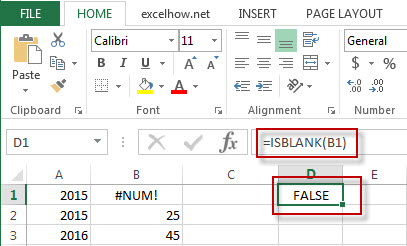### Related Functions

• Excel ISNUMBER Function
The Excel ISNUMBER function returns TRUE if the value in a cell is a numeric value, otherwise it will return FALSE.The syntax of the ISNUMBER function is as below:=ISNUMBER (value)
• Excel ISERR Function
The Excel ISERR function returns TRUE if the value is any error value except #N/A.The syntax of the ISERR function is as below:= ISERR (value)…
• Excel ISError Function
The Excel ISERROR function used to check for any error type that excel generates and it returns TRUE for any error type, such as: #N/A, #VALUE!,#REF!,#DIV0!, #NAME?, etc. The syntax of the ISERROR function is as below:= ISERROR (value)…
• Excel ISNA Function
The Excel ISNA function used to check if a cell contains the #N/A error, if so, returns TRUE; otherwise, the ISNA function returns FALSE. The syntax of the ISNA function is as below:=ISNA(value)…
• Excel ISTEXT Function
The Excel ISTEXT function used to check if a value is text. If so, returns TRUE; if the text is not text, the function will return FALSE. The syntax of the ISTEXT function is as below:=ISTEXT(value)…
• Excel ISNONTEXT Function
The Excel ISNONTEXT function used to check if a value is text. If so, returns FALSE; if the text is not text, the function will return TRUE. The syntax of the ISNONTEXT function is as below:=ISNONTEXT (value)…
• Excel ISREF Function
The Excel ISREF function used to check if a value is a valid reference. If so, returns TRUE; if the value is not a reference, the function will return FALSE. The syntax of the ISREF function is as below:=ISREF(value)…

## Excel Info Function

This post will guide you how to use Excel INFO function with syntax and examples in Microsoft excel.

### Description

The Excel INFO function returns information about the operating environment.

The INFO function is a build-in function in Microsoft Excel and it is categorized as an Information Function.

The INFO function is available in Excel 2016, Excel 2013, Excel 2010, Excel 2007, Excel 2003, Excel XP, Excel 2000, Excel 2011 for Mac.

### Syntax

The syntax of the INFO function is as below:

= INFO (type_text)

Where the INFO function arguments are:
Type_text -This is a required argument. The type of information that you want to return about the operating environment.

The following type values can be used:

 Type_text Returns “directory” Path of the current directory or folder. “numfile” Number of active worksheets in the open workbooks. “origin” Returns the absolute cell reference of the top and leftmost cell visible in the window, based on the current scrolling position, as text prepended with “\$A:”. This value is intended for for Lotus 1-2-3 release 3.x compatibility. The actual value returned depends on the current reference style setting. Using D9 as an example, the return value would be: ·         A1 reference style     “\$A:\$D\$9”. ·         R1C1 reference style    “\$A:R9C4” “osversion” Current operating system version, as text. “recalc” Current recalculation mode; returns “Automatic” or “Manual”. “release” Version of Microsoft Excel, as text. “system” Name of the operating environment: Macintosh = “mac” Windows = “pcdos”

From Microsoft website

### Example

The below examples will show you how to use Excel INFO Function to return the information about the operating environment.

#1 =INFO(“OSVERSION”)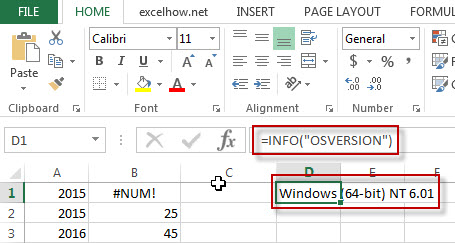Note: the above excel formula will return the current operating system version.

#2 =INFO(“system”)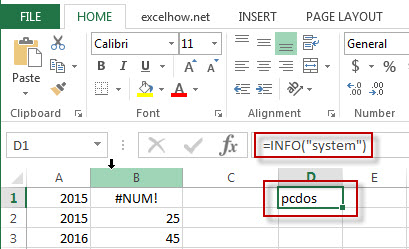Note: The above excel formula will return the name of the current operating environment.

## Excel Error.type Function

This post will guide you how to use Excel ERROR.TYPE function with syntax and examples in Microsoft excel.

### Description

The Excel ERROR.TYPE function returns a numeric value of one of the error type in EXCEL. If No error exists, the #N/A error will be returned.

The ERROR.TYPE function is a build-in function in Microsoft Excel and it is categorized as an Information Function.

The ERROR.TYPE function is available in Excel 2016, Excel 2013, Excel 2010, Excel 2007, Excel 2003, Excel XP, Excel 2000, Excel 2011 for Mac.

### Syntax

The syntax of the ERROR.TYPE function is as below:

= ERROR.TYPE (error_value)

Where the ERROR.TYPE function arguments are:
Error_value -This is a required argument.  The following error types can be used:

 If error_val is ERROR.TYPE returns #NULL! 1 #DIV/0! 2 #VALUE! 3 #REF! 4 #NAME? 5 #NUM! 6 #N/A 7 #GETTING_DATA 8 Anything else #N/A

### Example

The below examples will show you how to use Excel ERROR.TYPE Function to return the numeric representation of one of the error type.

#1 = ERROR.TYPE (B1)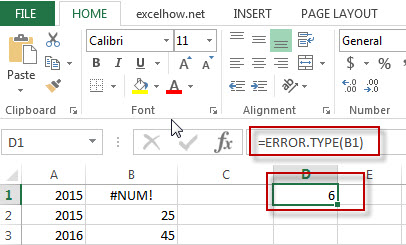Note: The above excel formula will return the numeric representation of “#NUM!” error type.

## Excel Cell Function

This post will guide you how to use Excel CELL function with syntax and examples in Microsoft excel.

### Description

The Excel CELL function returns information about the formatting, location, size, or contents of a cell.

The CELL function is a build-in function in Microsoft Excel and it is categorized as an Information Function.

The CELL function is available in Excel 2016, Excel 2013, Excel 2010, Excel 2007, Excel 2003, Excel XP, Excel 2000, Excel 2011 for Mac.

### Syntax

The syntax of the CELL function is as below:

= CELL (info_type,[reference])

Where the CELL function arguments are:
Info_type -This is a required argument. The type of information that you want to get. The following types can be used.

From Microsoft site:

 info_type Returns “address” Reference of the first cell in reference, as text. “col” Column number of the cell in reference. “color” The value 1 if the cell is formatted in color for negative values; otherwise returns 0 (zero). NOTE: This value is not supported in Excel Online, Excel Mobile, and Excel Starter. “contents” Value of the upper-left cell in reference; not a formula. “filename” Filename (including full path) of the file that contains reference, as text. Returns empty text (“”) if the worksheet that contains reference has not yet been saved. NOTE: This value is not supported in Excel Online, Excel Mobile, and Excel Starter. “format” Text value corresponding to the number format of the cell. The text values for the various formats are shown in the following table. Returns “-” at the end of the text value if the cell is formatted in color for negative values. Returns “()” at the end of the text value if the cell is formatted with parentheses for positive or all values. NOTE: This value is not supported in Excel Online, Excel Mobile, and Excel Starter. “parentheses” The value 1 if the cell is formatted with parentheses for positive or all values; otherwise returns 0. NOTE: This value is not supported in Excel Online, Excel Mobile, and Excel Starter. “prefix” Text value corresponding to the “label prefix” of the cell. Returns single quotation mark (‘) if the cell contains left-aligned text, double quotation mark (“) if the cell contains right-aligned text, caret (^) if the cell contains centered text, backslash (\) if the cell contains fill-aligned text, and empty text (“”) if the cell contains anything else. NOTE: This value is not supported in Excel Online, Excel Mobile, and Excel Starter. “protect” The value 0 if the cell is not locked; otherwise returns 1 if the cell is locked. NOTE: This value is not supported in Excel Online, Excel Mobile, and Excel Starter. “row” Row number of the cell in reference. “type” Text value corresponding to the type of data in the cell. Returns “b” for blank if the cell is empty, “l” for label if the cell contains a text constant, and “v” for value if the cell contains anything else. “width” Column width of the cell, rounded off to an integer. Each unit of column width is equal to the width of one character in the default font size. NOTE: This value is not supported in Excel Online, Excel Mobile, and Excel Starter.

### Example

The below examples will show you how to use Excel CELL Function to retrieve information about a cell.

#1 =CELL(“row”,B1)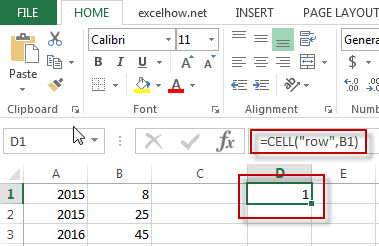Note: the above excel formula will return the row number of Cell B1.

#2 =CELL(“contents”,A1)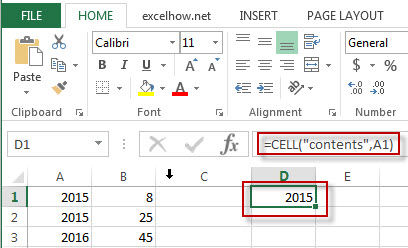Note: The above excel formula will return the content of cell A1.

#3 =CELL(“width”,B1)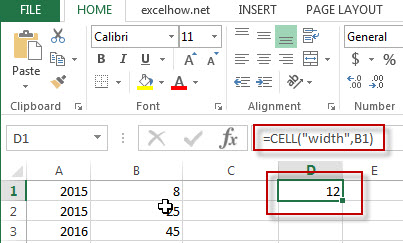Note: the above excel formula will return the column width of the cell B1.

### More CELL Function Examples

• Quickly Get Sheet Name
If you want to quickly get current worksheet name only, then inert it into one cell. You can use a formula based on the MID function in combination with the FIND function and the Cell function……
• Insert The File Path and Filename into Cell
If you want to insert a file path and filename into a cell in your current worksheet, you can use the CELL function to create a formual……..